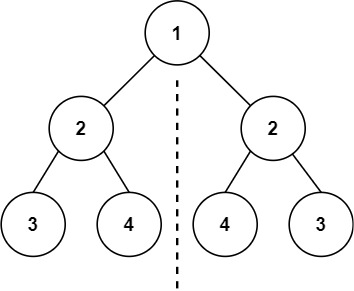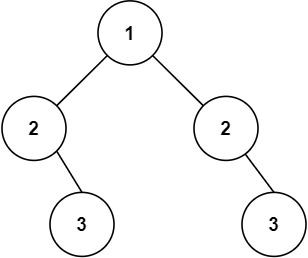## C# || How To Determine Whether A Binary Tree Is A Symmetric Tree Using C#The following is a module with functions which demonstrates how to determine whether a binary tree is a symmetric tree using C#.

1. Is Symmetric – Problem Statement

Given the root of a binary tree, check whether it is a mirror of itself (i.e., symmetric around its center).

Example 1:``` Input: root = [1,2,2,3,4,4,3] Output: true ```

Example 2:``` Input: root = [1,2,2,null,3,null,3] Output: false ```

2. Is Symmetric – Solution

The following is a solution which demonstrates how to determine whether a binary tree is a symmetric tree.

For two trees to be mirror images, the following three conditions must be true:

``` • 1 - Their root node's key must be same • 2 - The left subtree of left tree and right subtree of right tree have to be mirror images • 3 - The right subtree of left tree and left subtree of right tree have to be mirror images ```

``` 2. Is Symmetric - Solution C# // ============================================================================ // Author: Kenneth Perkins // Date: Oct 20, 2021 // Taken From: http://programmingnotes.org/ // File: Solution.cs // Description: Determines whether a binary tree is a symmetric tree // ============================================================================ /** * Definition for a binary tree node. * public class TreeNode { * public int val; * public TreeNode left; * public TreeNode right; * public TreeNode(int val=0, TreeNode left=null, TreeNode right=null) { * this.val = val; * this.left = left; * this.right = right; * } * } */ public class Solution { public bool IsSymmetric(TreeNode root) { return IsMirror(root, root); } private bool IsMirror(TreeNode a, TreeNode b) { if (a == null && b == null) { return true; } if (a == null || b == null) { return false; } // 1. The two root nodes have the same value // 2. The left subtree of one root node is a mirror // reflection of the right subtree of the other root node // 3. The right subtree of one root node is a mirror // reflection of the left subtree of the other root node return a.val == b.val && IsMirror(a.left, b.right) && IsMirror(a.right, b.left); } }// http://programmingnotes.org/ 123456789101112131415161718192021222324252627282930313233343536373839404142 // ============================================================================//    Author: Kenneth Perkins//    Date:   Oct 20, 2021//    Taken From: http://programmingnotes.org///    File:  Solution.cs//    Description: Determines whether a binary tree is a symmetric tree// ============================================================================/** * Definition for a binary tree node. * public class TreeNode { *     public int val; *     public TreeNode left; *     public TreeNode right; *     public TreeNode(int val=0, TreeNode left=null, TreeNode right=null) { *         this.val = val; *         this.left = left; *         this.right = right; *     } * } */public class Solution {    public bool IsSymmetric(TreeNode root) {        return IsMirror(root, root);    }     private bool IsMirror(TreeNode a, TreeNode b) {        if (a == null && b == null) {            return true;        }        if (a == null || b == null) {            return false;        }        // 1. The two root nodes have the same value        // 2. The left subtree of one root node is a mirror        // reflection of the right subtree of the other root node        // 3. The right subtree of one root node is a mirror        // reflection of the left subtree of the other root node        return a.val == b.val &&            IsMirror(a.left, b.right) &&            IsMirror(a.right, b.left);    }}// http://programmingnotes.org/ ```

QUICK NOTES:
The highlighted lines are sections of interest to look out for.

The code is heavily commented, so no further insight is necessary. If you have any questions, feel free to leave a comment below.

Once compiled, you should get this as your output for the example cases:

``` true false ```